RUSSIAN JOURNAL OF EARTH SCIENCES, VOL. 12, ES5004, doi:10.2205/2012ES000523, 2012

# A new technique for recognizing fractured zones in P-P reflection fields, applied to the study of a North Sea oil reservoir

Irina I. Semerikova1, J. Russ Evans2, David C. Booth2, Hengchang Dai2, Tatiana S. Blinova1

1Organization of the Russian Academy of Sciences Mining Institute of the Ural Branch of the RAS, Perm, Russia

2British Geological Survey, Edinburgh, UK

.

### Abstract

A technique is presented for recognizing different types of fractured zones within the internal subsurface structure, including zones of extended fractures with dimensions larger than the signal wavelength, and zones of small differently oriented fractures with dimensions smaller than the signal wavelength. The technique is based on dynamic analysis, using prospecting indicators for fractures and faults of different classes, determined from the dynamic parameters of P-P reflections. These prospecting indicators are determined from the results of seismic modeling, as well as physical modeling with samples of natural rock and field seismic surveys on known fractured objects with fracture parameters in different lithologic rock types. We have studied the quality, stability and statistical significance of the indicators, and on the basis of the dynamic analysis we determine the probability of the presence of fractured objects of a particular type. The resulting technique has been applied to a fractured hydrocarbon reservoir under the North Sea, where it has been possible to better determine the particular features of its geologic structure. Fractured zones in a gas-saturated unstratified subsurface have been mapped; possible large tectonic faults and block structure have been revealed; results show the likely presence of diffracting objects within the rock matrix, determine their type, and improve the imaging of reflecting horizons with weak intensity and fragmented correlation.

### 1. Introduction

One of the basic research problems of seismic prospecting is the exploration and mapping of fractured zones. We obtain additional information about faults and fractures on the basis of studying changes of dynamic parameters (amplitude, spectra) of the P-P reflection. It is expected that for fractured zones with different internal structures the prospecting indicators should be different, and primarily the dynamic characteristics of seismic waves, as they are the most sensitive to changes in geological factors. It is clear that this seismic prospecting problem is not a simple one, due to the many factors which influence the dynamic parameters [Ampilov, 2004; Blias, 2005], as well as their smaller resistance to noise caused by signal reception and processing, when compared with the kinematic parameters. However in recent years dynamic parameters have become increasingly attractive for the interpreter, when they bring new information on features of the subsurface, due to their sensitive reaction to its seismic response, together with rapidly appearing new techniques directed to preserving the validity of their values during field data processing.

Since the inverse problem of seismic prospecting is generally ambiguous, the majority of interpretive methods use empirically-found connections between seismic parameters and the physical properties of the subsurface.

Our investigations in recent years [Babkin and Akhmatov, 2004; Semerikova, 2002, 2005a, 2005b, 2006b] have been aimed at finding connections between the physical-mechanical properties of the subsurface (including fractures) and the set of seismic dynamic parameters, in order to identify and map anomalies. For this, a detailed survey has been performed to find the most informative and stable dynamic parameters. We studied their behavior and the degree of sensitivity, which are important features of their dependence on the fracturing parameters.

### 2. Determination of Dynamic Parameters

The problem of determining the set of dynamic parameters for fractured objects of different types and classes has been solved by using three different and independent methods [Semerikova, 2002, 2005a, 2005b].

1. Mathematical modelling of the theoretical wave fields was performed using synthetic seismogram software based on ray theory. Modelling was carried out for 67 different models, with and without fractures of various types:

• single inclined quasi-vertical, with dimensions either much larger or smaller than the signal wavelength, or comparable to the signal wavelength, slightly less than the signal wavelength;
• an accumulation of small fractures (with dimensions much smaller than the signal wavelength) in different lithologic layers. For seismic modelling, we use realistic parameters defining the elastic properties, lithologic and structural characteristics of the subsurface, defined by geological and geophysical data in field areas (Volga-Ural Oil Province, Permskoye Prikamje, Verkhnekamskoye Potassium Salt Deposit).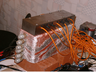Figure 1

2. Research was also performed using physical modeling. The acquisition geometry for common depth points (CDP) [Babkin and Akhmatov, 2004; Sanfirov, 1999] was simulated on natural rock samples (parallelepipeds $30\times 20 \times 10$ cm, see Figure 1). Shooting is conducted along the two orthogonal surfaces of the samples – on a plane parallel to the horizontal layering of the samples and in a plane of vertical layering. Measurements are performed by geophones, and the excitation source is a bolt hit by a hammer. Experiments are performed in the high-frequency range (HF filter 200 Hz – LF filter 20 kHz).

Different fracture models were used. In the experiments, the rock samples were sawn through to create fractures. The fracture models include:

• single oblique fracture longer than the signal wavelength;
• two non-parallel fractures one of which is smaller than the signal wavelength;
• cylindrical hole;
• different single-axial loadings on samples without fractures, aimed at studying the behavior of dynamic parameters under changes of stress-deformation [Semerikova, 2007a, 2011].

3. Parameters were also investigated and derived by using seismic CDP in different regions where there are "training objects" – fractured bodies with known geology and fracture parameters – in naturally occurring different lithologic rock types (terrigeneous, carbonate, evaporites). An example is the extended single fractures and accumulations of small differently oriented fractures, opened and recorded during mine excavations in the Verkhnekamskoye Potassium Salt Deposit. In this case, the mine seismic data have been used. Other training objects, confirmed by wells, are: fractured oil reservoirs in the Western Urals Folding zone, and in the Pre-Urals depression in the Tournaisian limestone in the anticlinal structures over Frasnian-Famennian reefs (Volga-Ural Oil Province); terrigenous deposits in the unique fractured reservoir of Bazhenov Formation in West Siberia, as well as fixed karst phenomena on the surface and in a near-surface zone in the areas of intensive development of sulphate-carbonate karst near Kungur, Perm region.

### 3. Prospecting Indicators

As a result of the above research studies, we have determined the prospecting indicators for fractured objects in the following dynamic parameters of P-P reflections [Semerikova, 2002, 2005a, 2005b]:

• mean amplitude $A_{\rm cp}$;
• frequency centroid coordinate $F_{\rm c}$ (where the centroid is the mathematical expectation of the amplitude spectrum);
• spectrum width;
• upper and lower frequency limits;
• gradients of the mean amplitudes in the directions of $X$ and $t$: grad$_X$.$A_{\rm cp}$, grad$_t$.$A_{\rm cp}$;
• difference of the amplitude spectra in intervals containing anomalous parameters and those which do not contain anomalies: $\Delta A(f)$;
• absolute values of parameters.

The parameters accepted as prospecting indicators are selected from the set of dynamic parameters (based on amplitude, spectra), as being the most stable and statistically significant, and where parameter anomalies exceed the noise level.

The differences in their variability allow distinguishing zones of different internal structure [Semerikova, 2005a, 2005b]. These prospecting indicators are grouped according to types (classes): for extended fractures (lengths greater or equal to signal wavelengths) and concentrations of small differently oriented fractures (lengths shorter than signal wavelengths).

#### 3.1. Prospecting Indicators for Extended Fractures

Amplitude indicators:

1. On a section of mean amplitudes, an inclined seismic event of large maximal values is formed. It is caused by the reflected wave from the fracture surface. This wave has the greatest intensity.
2. The reflected wave from the surface of the inclined fracture is shown by abnormally high absolute values of the gradients of the mean amplitudes in the direction of $t$.
3. On the reflecting horizons under an inclined fracture, the values of mean amplitudes tend to zero within a segment of dimension equal to the projection of the fracture at these horizons. A "shadow" segment is formed.
4. On sections of absolute values of mean amplitudes the zero values represent a "shadow segment" in a shape of a triangle formed by the fracture surface and its projection on the underlying horizon near by the lower end of the fracture.
5. The "shadow segment" under a vertical fracture is transformed into a trough or peak, showing zero values of mean amplitudes directly at the lower end of the fracture.
6. The intersection area of the reflecting horizon with both vertical and inclined fractures is identified by extreme values of mean amplitudes of the reflecting horizon.
7. There exist high absolute values of the gradient of mean amplitudes of the reflecting horizon in the area of a fracture.
8. A trough (minimum value) of the mean amplitude of the reflecting horizon exists at the upper end of a fracture. This minimum value is half or less of the mean amplitude.

Spectral indicators:

1. A maximum decrease of the spectral composition of the elastic waves in the time interval containing a fracture occurs on the horizontal area of length equal to the projection of inclined fracture to the land surface. There happens a scattering of high-frequency elastic waves, whose wavelength is commensurate with the length of the projection of the fracture. The active band shifts towards low frequencies and so the frequency coordinate of the centroid also shifts.
2. Decreasing frequency of the maximum of the amplitude spectrum occurs on the horizontal area of length equal to the projection of inclined fracture to the land surface.
3. For a vertical fracture, a reduction in the frequency spectral peak occurs just at the intersection with the reflecting horizon.
4. An abrupt, intermittent increase and decrease of the spectral amplitudes occurs when the seismic profile intersects the vertical fracture. A steady relaxation of the spectral amplitudes takes place with increasing distance from the fracture.
5. For inclined fractures, the values of the amplitudes of the spectra on both sides of the fracture are very different.
6. The horizontal extent of the anomaly of the maximum difference between the energy spectra in the interval without a fracture and the interval containing the fracture is equal to the length of the inclined fracture projection at the reflecting horizon. The values of the frequency coordinate of the centroid, for this anomaly, diminish when the length of the fracture projection increases. Thus, there is scattering of waves whose wavelength is similar to the lengths of the fracture projection.
7. There exist high absolute values of the gradient of mean amplitudes of the reflecting horizon in the area of a fracture.
8. A trough (minimum value) of the mean amplitude of the reflecting horizon exists at the upper end of a fracture. This minimum value is half or less of the mean amplitude.

#### 3.2. Prospecting Indicators for Zones of Concentration of Small Differently Oriented Fractures

1. In fractured zones – the absolute values of mean amplitudes are zero (or close to zero).
2. On the sections of mean amplitudes there are no inclined seismic events existing in the presence of extended inclined fractures.
3. In the spectral parameters sections (i.e. frequency coordinate of the centroid, frequency of the maximum of the amplitude spectrum) a maximum increase of frequency values is found in the area of the zones of concentration of small differently oriented fractures. This is in contrast to the decrease in these parameters which is characteristic of the extended fractures.
4. In favourable conditions, the maximum gradient of the mean amplitudes may image the "smooth" walls of the fractured zone.
5. Zero values in the section of the module of a gradient of the mean amplitudes over time indicate the zones of small differently oriented fractures, whose combination can create diffracting objects.
6. The horizontal extent of the anomaly of the maximum difference between the energy spectra in the interval without a fracture and the interval containing the fracture is equal to the length of the inclined fracture projection at the reflecting horizon. The values of the frequency coordinate of the centroid, for this anomaly, diminish when the length of the fracture projection increases. Thus, there is scattering of waves whose wavelength is similar to the lengths of the fracture projection.
7. There exist high absolute values of the gradient of mean amplitudes of the reflecting horizon in the area of a fracture.
8. A trough (minimum value) of the mean amplitude of the reflecting horizon exists at the upper end of a fracture. This minimum value is half or less of the mean amplitude.

### 4. Technique for Recognition of Fracture Zones

Application efficiency is based on the objective preconditions. They are the following:

• the occurrence of certain anomalies of amplitude and spectral parameters, caused by the presence of fractures of various types;
• a display of regular matches in the behaviour of the given parameter anomalies;
• anomalies must exceed the level of noise and the errors remaining after compensation for other factors which influence the given parameters [Blias, 2005].
• In favourable conditions, the maximum gradient of the mean amplitudes may image the "smooth" walls of the fractured zone.
• Zero values in the section of the module of a gradient of the mean amplitudes over time indicate the zones of small differently oriented fractures, whose combination can create diffracting objects.
• The horizontal extent of the anomaly of the maximum difference between the energy spectra in the interval without a fracture and the interval containing the fracture is equal to the length of the inclined fracture projection at the reflecting horizon. The values of the frequency coordinate of the centroid, for this anomaly, diminish when the length of the fracture projection increases. Thus, there is scattering of waves whose wavelength is similar to the lengths of the fracture projection.
• There exist high absolute values of the gradient of mean amplitudes of the reflecting horizon in the area of a fracture.
• A trough (minimum value) of the mean amplitude of the reflecting horizon exists at the upper end of a fracture. This minimum value is half or less of the mean amplitude.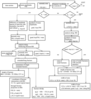Figure 2

The criteria of the statistical significance of an anomaly are the root-mean-square error of parameter values $\delta$, and the value $1/4 - 1/6$ $D$, where $D$ is the diameter of the first Fresnel zone. The algorithm for the interpretation (and recognition) of fractured zones within internal structure involves a two-step scheme (Figure 2). Methodically the analysis of parameters begins with the universal prospecting indicators in the P-P reflection fields, which are significant for all different classes of fractured zones. At the second stage a transition to individual, unique indicators and more specific problems is carried out. As a result it is obviously possible to reveal and make a division of fractured zones according to their internal structure. One of the branches of the scheme deduces the zones of small differently-oriented fractures, another deduces the extended fractures. Our approach to the solution is to partition it into three tasks.

The initial task is to obtain certain differential dynamic parameters in the form of time sections. This is performed in the framework of our technique of dynamic analysis for each time section value.

The second task is to find in sections some certain anomalies in the behaviour of these dynamic parameters caused by occurrence of a fractured body of some class. These anomalies must exceed the noise level remaining after processing. Then the credibility level of the existence of these anomalies is estimated on the basis of the determination of errors remained after compensation for other factors affecting these seismic parameters.

For each time reading we can write an equation for the approximate coefficient of probability of the existence of anomaly of a dynamic parameter. We present a formula in the following way

\begin{eqnarray*} r_k (t_i, x_j) = \mbox{ if } \, ((c(t_i, x_j) - c_{\rm cp} \geq n_1 \delta \end{eqnarray*}

\begin{equation} \tag*{(1)}\label{1} \mbox{ if } \, ((c(t_i, x_j) - c_{\rm cp} \geq n_2 \delta); 1; 0.5) ;0 \end{equation}

where $c(t_i, x_j)$ is a parameter value at a point on the time section, $c_{\rm cp}$ is its mean value in the interval of the time section, $\delta$ is the RMS deviation of the parameter, $i$, $j$ – sample number, trace number, $n_1$, $n_2$ – known coefficients depending on quality of seismic data.

The final task is the formation of a time section of the effective parameter of the presence probability of the fractured body. After the evaluation of the probabilities of presence of anomalies of parameters they are converted into a common parameter of probability of fractured object presence of some special type (extended or small fractures) – the effective parameter. This parameter is the main output parameter. Estimation of the probability of the presence of a certain class of object is realized through effective accumulation (summation) of all evaluations of reliability of seismic parameters anomalies. Effective parameter of probability

\begin{equation} \tag*{(2)}\label{2} c_{pp} (t_i, x_j) = \sum_{1}^k a_k r_k (t_i, x_j) \end{equation}

where $a_k$ is the known intensity of the $k$-th dynamic parameter (or weight).

### 5. Application: A Case Study From the North Sea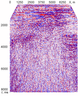Figure 3

This work was carried out as part of a collaboration between the Mining Institute of the Urals Branch of Russian Federation Academy of Sciences (UB RAS) and the British Geological Survey (BGS). The study is conducted on a geological site in the North Sea. The initial data were a seismic stacked section of P-waves, provided by the Kerr McGhee oil company to the British Geological Survey (BGS) (Figure 3). The aim is to study the possibilities of extracting additional information about the disjunctive dislocations within a large unstratified anticline applying the technique of recognizing of fractured zones in the P-P reflection wavefield, as developed by the Mining Institute, UB RAS, based on analysis of the dynamic (amplitude indicators, frequency parameter) characteristics of elastic waves [Semerikova, 2006a, 2006b, 2007a].

Our idea was to develop aspects of our technique, as the use of standard seismic methods for layered media is not possible for mapping fractured zones in that type of body. One reason for this is that stable reflectors within the given body are not recorded. The technique is based on prospecting indicators, determined from the dynamic parameters of the P-P reflection for the fractured bodies [Semerikova, 2002, 2005a, 2005b], as described in the previous sections.

The technique for recognizing fractured objects is based on the calculation of parameter-indicators for seismic time sections.

A detailed study of the variability of the behavior of the fracture indicators gives a deeper understanding of the relationships between the effective dynamic parameters of the P-P reflection and the characteristics of fractures and faults – that is, any dislocation causing a break of continuity. This can give new information, especially in the presence of different types of dislocation. We illustrate this using some parameters calculated for the geological body in the North Sea.

In the center of the stacked section (Figure 3). one can see an unstratified body with no stable reflections inside. In the upper part of the anticline there are buried focus reflections, which occur when the curvature of the synclinal reflector exceeds the curvature of the wavefront. Their presence masks structural characteristics of reflectors in the corresponding time interval, i.e. in the interval 2000–2400 ms at CDP location $= 4350-5000$ m. This small part of the section will not be considered in the analysis. All the rest of the section is analyzed.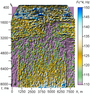Figure 4

The interpretation is carried out by integrated study of all received calculations of the dynamic parameters. Besides the main output parameters we analyzed significant information contained in separate dynamic parameters included as output parameters for a certain class (type) of fractured objects [Semerikova, 2002, 2005b, 2007b, 2008]. For example, the section of the spectral parameters (frequency coordinate of the centroid) clearly indicates the boundaries of the investigated unstratified "neck-shaped" anticline (Figure 4).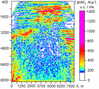Figure 5

In investigating the internal structure of the neck-shaped anticline, we suggest that the zones of zero absolute values of the derivative of the mean amplitudes with $t$ (shown in Figure 5) may indicate the presence of diffracting objects. An example of this type of object is a hole, with a diameter of ten or more times smaller than the wavelength of the used signal. The basis for this hypothesis is provided by the following results of seismic investigations on a rock sample [Semerikova, 2007a, 2007b], on which the acquisition geometry for the CDP method is simulated. It is the same acquisition geometry which is commonly applied at Verkhnekamsk mine over single mine workings [Babkin and Akhmatov, 2004; Sanfirov, 1999; Semerikova, 2005a, 2006b, 2007a].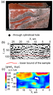Figure 6

In a parallelepiped rock sample of size $34 \times 16 \times 11$ cm a cylindrical hole was drilled through its entire thickness, and perpendicular to a CDP reflection profile. The CDP reflection profile passed over the upper face of the parallelepiped (Figure 6). The zero absolute values of the derivative of the mean amplitudes with t identified the location of the hole. This physical experiment proves that similar anomalies of this dynamic parameter may be caused by diffraction. Analogously, we may assume the presence of such diffracting elements in the internal structure of this rock matrix.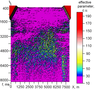Figure 7

The main output parameters of the technique are the effective parameters, evaluating the probability of the presence of fractured zones of any type (extended or small). Figure 7 shows the distribution pattern of the effective parameter (equation 2), evaluating the probability of the presence of extended fractures (of length greater than the signal wavelength).

The location of the highest concentration of high probability zones between $T= 2400$ and 5600 ms and $X=3750$ and 6250 m coincides with the known (a priori information) position of gas clouds in the "neck-shaped" end of the anticline. Gas clouds are indicators of fractures in the body. Some of these zones are located in the lower time interval, beneath the "neck" in the body of the unstratified anticline. Subvertical narrow zones of possible extended dislocations with a break of continuity are observed on the left and right of the structure, which create an impression of block structure.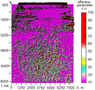Figure 8

The distribution of the effective parameter of the presence probability of zones of small differently oriented fractures within the unstratified body is widely dispersed (Figure 8). It should be noted that these effective parameters identifying fractured zones of different types show results with a chaotic orientation of fractures as well, i.e. when the problem of finding these areas is difficult for methods based on azimuthal analysis (for example, AVO).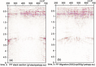Figure 9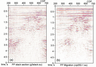Figure 10

One of the main problems for Dai et al.  and Li et al. , was to display the reflectors in a relatively shallow dome-shaped structure placed beneath the "neck" at $t= 3200-3600$ ms in Figure 3. Dai et al.  used Prestack Kirchoff Time Migration (PKTM) and transformation of PS-waves (Figure 9. As gas clouds "soften" the P-waves, the PKTM technique applied to P-waves did not give an effective result (Figure 10. The PKTM approach also takes time and requires long PC processing. Besides, the field geometry required for recording PS-waves is usually an additional and expensive procedure.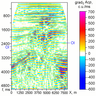Figure 11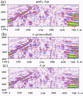Figure 12

One of the advantages of the application of our recognition technique for fractured zones to PP reflections is that the display and behavior of the reflectors in this anticlinal structure are clearly expressed in the sections of gradient of mean amplitudes (Figure 11 and Figure 12).

Moreover in some cases, reflectors like reflector "OI" in Figure 11 are clearly evident. Usually in both stacked sections of P and S waves (Figure 9 and Figure 10), either they cannot be correlated because of their weak intensity, or it is only possible to map them fragmentarily. Sections of gradients of mean amplitudes allow us to identify them at both sides of the unstratified anticline (Figure 11).

The results of such studies of the behavior of the dynamic parameters occupy an intermediate position in the recognition techniques for fractured zones and form a basis for using the adaptation algorithm of this technique for a "seismically transparent" medium, i.e. for a medium without correlated reflectors. It is obvious that the technique presented here may be efficiently involved in the interpretation process. Effective results can be obtained for the problem of evaluating the character of fractures of different types. The revealed features in the behavior of the dynamic characteristics of P-waves play a role in identifying the geological origins of these wavefield anomalies.

### 6. Conclusions

In summary, the following additional information was obtained for the geological body in the North Sea:

1. The application of this technique allows the detection and mapping of zones of extended fractures of length greater than the signal wavelength. Moreover, their spatial location is mapped "directly". Such mapping cannot be done with classical standard methods: disruption of a seismic event suggests a thrust-plane or slip plane, but the presence of associated fracturing is only a guess, and it cannot be imaged. Furthermore, the results of this technique allow the mapping of fracturing with no dominant azimuthal direction. This type of fracturing cannot be identified with azimuthally oriented methods such as AVO, and the AVO-method did not reveal the presence of fracturing on the object [Dai et al., 2007]. The spatial location of such zones is confirmed by the spatial position of the gas clouds. Moreover, the spatial position of the zones of extended fractures suggests a block structure for the object.
2. Similar results are obtained for the presence of zones of small differently-oriented fractures.
3. The results suggest the existence of many diffracting elements like cylindrical holes in the body of the studied object, the location of which corresponds to the location of the gas cloud.
4. Finally, a variety of dynamic parameters allow us to obtain a bright image of reflecting horizons with weak intensity and fragmented correlation, and to perform more reliable correlation of similar reflecting horizons.
5. Zero values in the section of the module of a gradient of the mean amplitudes over time indicate the zones of small differently oriented fractures, whose combination can create diffracting objects.
6. The horizontal extent of the anomaly of the maximum difference between the energy spectra in the interval without a fracture and the interval containing the fracture is equal to the length of the inclined fracture projection at the reflecting horizon. The values of the frequency coordinate of the centroid, for this anomaly, diminish when the length of the fracture projection increases. Thus, there is scattering of waves whose wavelength is similar to the lengths of the fracture projection.
7. There exist high absolute values of the gradient of mean amplitudes of the reflecting horizon in the area of a fracture.
8. A trough (minimum value) of the mean amplitude of the reflecting horizon exists at the upper end of a fracture. This minimum value is half or less of the mean amplitude.

#### Acknowledgments

We thank our various colleagues who provided the seismic materials which were used to apply the recognition technique reported here, especially Xiang-Yang Li and Zhongping Qian (BGS), Vladimir Vanyukhin ("Surgut TISIZ" Co. LTD), and the Kerr McGhee oil company. We also thank Irina Shibkova who assisted us by translating our more complex discussions. This paper is published with the permission of the Executive Director of the British Geological Survey (NERC) and the Director of the Mining Institute of the Ural Branch of the Russian Academy of Sciences.

### References

Ampilov, Y. P. (2004), Seismic interpretation: experience and problems, Geoinformation, Moscow.

Babkin, A. I., and A. E. Akhmatov (2004), Capability assessment of the technique of multiple coverages for mapping an isolated dislocation with a break of continuity on the basis of physical modelling. Strategy and processes of geological resources development. Summary reports, Mining Institute of UB RAS, Perm.

Blias, E. A. (2005), Linear approach to define impedances of compressional and shear waves by seismograms of P-reflection. (AVO-inversion), Technology of seismic exploration, 1, 5–15.

Dai, H., X.-Y. Li, P. Conway (2007), Imaging beneath gas clouds using 3D prestack Kirchhoff time migration of PS-converted waves – a case study from the North Sea, The Leading Edge 26, 522–529.

Li, X.-Y., H. Dai, F. Mancini (2007), Converted-wave imaging in anisotropic media: theory and case studies, Geophysical Prospecting, 55, 345–363.

Sanfirov, I. A. (1999), Mining problems of seismic exploration by the CDP method, UB RAS, Ekaterinburg.

Semerikova, I. I. (2002), Parameter estimation of fracture development zones with seismic exploration data. Problems of complex monitoring in the fields of mineral resources, Summary reports, Mining Institute of UB RAS, Perm, 90–95.

Semerikova, I. I. (2005a), Dynamic characteristics of seismic waves for the solution of geological problems in fractured media. Strategy and processes of geological resources development, Records of the scientific session of Mining Institute of UB RAS for 2004, Mining Institute of UB RAS, Perm, 138–142.

Semerikova, I. I. (2005b), A technique for recognizing fractured media in P-P reflection wavefields, Proceedingsof the VII International Workshop Conference "Geomodel-2005", Gelendzhik, 97.

Semerikova, I. I. (2006a), Aspects of techniques for recognizing fractured media by the analysis of complex wavefields. Strategy and processes of geological resources development, Records of the scientific session of Mining Institute of UB RAS for 2005, Mining Institute of UB RAS, Perm, 261–263.

Semerikova, I. I. (2006b), Expanding the spectrum of techniques for recognizing fractured media by analysis of dynamic parameters of seismic waves, Proceedings of the VIII International Workshop Conference "Geomodel-2006", Gelendzhik, 2006.

Semerikova, I. I. (2007a), Display of the stress-deformed status of rocks in the dynamic parameters of seismic waves. Strategy and processes of geological resources development, Records of the scientific session of Mining Institute of UB RAS for 2006, Mining Institute of UB RAS, Perm, 217–219.

Semerikova, I. I. (2007b), Application of a technique for recognizing fractured media in studying transformation of the stress-deformed status of rocks, Proceedingss of the IX International Workshop Conference and Exhibition "GEOMODEL-2007", Gelendzhik, 28–29.

Semerikova, I. I. (2008), Seismic approach for studying breaking of the rock mass in zones of extended fractures. Strategy and processes of geological resources development, Records of the scientific session of Mining Institute of UB RAS for 2007, 124–126.

Semerikova, I. I. (2011), Potential of the technique of recognition of the fractured zones on seismic parameters for study of technogenic changes of the state of the rocks, Mining Informational and Analytical bulletin, Mining Book, Moscow.

Received 15 September 2012; accepted 17 September 2012; published 29 October 2012.Citation: Semerikova Irina I., J. Russ Evans, David C. Booth, Hengchang Dai, Tatiana S. Blinova (2012), A new technique for recognizing fractured zones in P-P~reflection fields, applied to the study of a North Sea oil reservoir, Russ. J. Earth Sci., 12, ES5004, doi:10.2205/2012ES000523.

Generated from LaTeX source by SemTeXML, v.1.2) software package.